# 18. Driven Oscillator

Michael Fowler (closely following Landau para 22)

Consider a one-dimensional simple harmonic oscillator with a variable external force acting, so the equation of motion is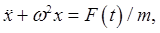which would come from the Lagrangian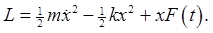(Landau "derives" this as the leading order non-constant term in a time-dependent external potential.)

The general solution of the differential equation is, where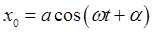, the solution of the homogeneous equation, and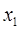is some particular integral of the inhomogeneous equation.

An important case is that of a periodic driving forceA trial solutionyields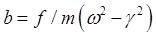soBut what happens when? To find out, take part of the first solution into the second, that is,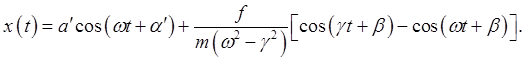The second term now goes to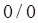as, so becomes the ratio of its first derivatives with respect to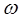(or, equivalently,).The amplitude of the oscillations grows linearly with time. Obviously, this small oscillations theory will crash eventually.

But what if the external force frequency is slightly off resonance?

Then (real part understood)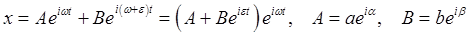with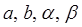real.

The wave amplitude squared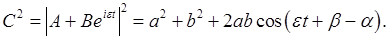We're seeing beats, with beat frequency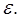Note that if the oscillator begins at the origin,then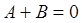and the amplitude periodically goes to zero, this evidently only occurs whenEnergy is exchanged back and forth with the driving external force.

## More General Energy Exchange

We'll derive a formula for the energy fed into an oscillator by an arbitrary time-dependent external force.

The equation of motion can be writtenand defining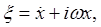this is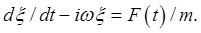This first-order equation integrates to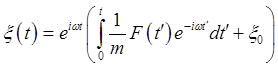The energy of the oscillator is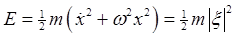So if we drive the oscillator over all time, with beginning energy zero,This is equivalent to the quantum mechanical time-dependent perturbation theory result: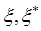are equivalent to the annihilation and creation operators.

## Damped Driven Oscillator

The linear damped driven oscillator: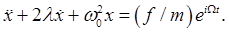(Following Landau's notation here -- note it means the actual frictional drag force is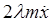)

Looking near resonance for steady state solutions at the driving frequency, with amplitude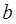, phase lag, that is,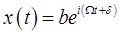, we findFor a near-resonant driving frequency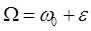, and assuming the damping to be sufficiently small that we can drop the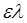term along with, the leading order terms give,

so the response, the dependence of amplitude of oscillation on frequency, is to this accuracy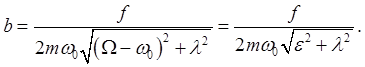(We might also note that the resonant frequency is itself lowered by the damping, but this is another second-order effect we ignore here.)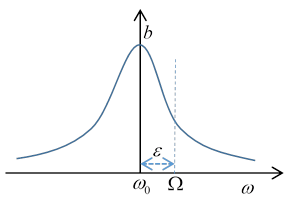The rate of absorption of energy equals the frictional loss. The friction force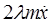on the mass moving at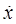is doing work at a rate: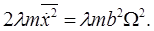The half width of the resonance curve as a function of driving frequency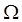is given by the damping. The total area under the curve is independent of damping.

For future use, we'll write the above equation for the amplitude as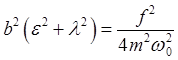.

The Excel spreadsheet for a driven damped oscillator I showed in class can be downloaded here.